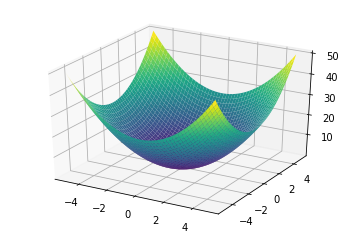A draft notebook from my Calculus II files.

In :
from sympy import *

In :
init_printing()


Consider:

$$f(x, y) = x^2 \sin{y}$$
In :
x, y = symbols('x y')

In :
f = x**2 * sin(y)


## Compute partial derivatives¶

In :
dx = diff(f, x)
dx

Out:
$$2 x \sin{\left (y \right )}$$
In :
dy = diff(f, y)
dy

Out:
$$x^{2} \cos{\left (y \right )}$$

## Nabla $\nabla$, "the gradient"¶

Also pronounced "del".

The gradient of $f$ is a vector that has all the partial derivatives in it.

In this case, $f$ is a two-variable function so there are two derivatives in it.

\begin{align} \nabla f &= \left[ \frac{f_x}{f_y} \right] \\ \\ &= \left[ \frac{ 2x \sin{y} }{ x^2 \cos{y} } \right] \end{align}

# Direction of steepest ascent¶

The gradient points in the direction of steepest ascent. Not 100% sure what this means...

## New function $g$¶

To match the example in the video linked above.

In :
g = x**2 + y**2
g

Out:
$$x^{2} + y^{2}$$
In :
g = plotting.plot3d(g, (x, -5, 5), (y, -5, 5))## $\nabla g$¶

In :
gradient = [
diff(g, x),
diff(g, y)
]


Out:
$$\left [ 2 x, \quad 2 y\right ]$$

The gradient is a function that takes in an $(x, y)$ input and returns a vector. Perhaps this vector points in the direction of steepest ascent?

## Direction of steepest ascent at $(1, 1)$¶

In :
p1 = {'x': 1, 'y': 1}

In :
delAtP1 = [d.subs(p1) for d in gradient]
delAtP1

Out:
$$\left [ 2, \quad 2\right ]$$

I think this means that if you are standing at $(1, 1, 2)$, you should "walk" in the $(2, 2)$ direction in order to reach a higher output value (since this is the fastest way). I don't really understand what this means.